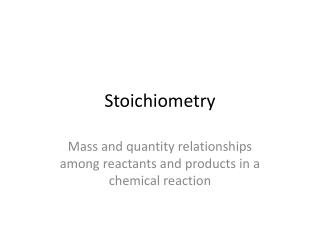DownloadDownload PresentationStoichiometry

# Stoichiometry

Télécharger la présentation## Stoichiometry

- - - - - - - - - - - - - - - - - - - - - - - - - - - E N D - - - - - - - - - - - - - - - - - - - - - - - - - - -
##### Presentation Transcript

1. Stoichiometry Mass and quantity relationships among reactants and products in a chemical reaction

2. Stoichiometry • Stoichiometry is the calculation of quantities of substances involved in chemical reactions. • Balanced chemical equations, through their coefficients, indicate the ratio of moles of reactants and products: • N2 + H2 → NH3 • N2 + 3 H2 → 2 NH3

3. Method for Solving Stoichiometry Problems • What am I looking for? • What do I know? • What’s my plan? • Let’s do it! • Units / Sig Figs

4. Mole-Mole Calculations • Given the moles of one reactant/product, calculate the moles required/produced of the other reactant/product • Steps to Follow: • Write the balanced chemical equation • Write the moles of the given reactant/product • Use the mole ratio (determined from balanced equation) in a conversion factor to calculate the amount of moles of desired reactant/product

5. 1st Stoichiometry ProblemMole-Mole Relationships • In a space station, carbon dioxide exhaled by astronauts can be removed by a reaction with lithium hydroxide. This reaction produces lithium carbonate (Li2CO3) and liquid water (which may be used for other productive purposes on the space station). • How many moles of lithium hydroxide would be needed to remove 20.0 mol of CO2 (the average daily amount exhaled by a human)? • How many moles of water are produced daily from this reaction

6. Mole Ratio Relates Products & Reactants

7. 2nd Stoichiometry ProblemMass-Mole Relationships • When magnesium burns in air a combustion reaction produces solid magnesium oxide. How many grams of magnesium oxide would be produced from 2.00 mol of magnesium?

8. Mole Ratio Relates Products & Reactants

9. 3rd Stoichiometry ProblemMass-Mass Relationships • Tin (II) fluoride (SnF2) is added to toothpaste to help prevent cavities. It is made by the single replacement reaction of tin with gaseous hydrogen monofluoride (HF). How many grams of tin (II) fluoride are produced from 30.00 g of hydrogen monofluoride?

10. Mole Ratio Relates Products & Reactants

11. Mole Ratio Relates Products & Reactants